# Top 20 Excel Limitations that might Frustrate You!

Excel is obviously one of the most important products in the world. It is very helpful in managing, analyzing data. But there is also something that may get us frustrated when using Excel. Today I’d like to share with you some frustrating issues and how to solve them.

## 1. Data cannot be displayed as you want

Suppose that you want to display “2-1” representing 1st Feb in Excel, what will you get after you enter “2-1” into Excel? Unfortunately, you will get “1-Feb” as shown in Figure 1. At this time, the method of formatting cells may come to our mind. But if we really open the Format Cells dialog box and click on Date, you will find that all formats available in Type field are useless in our case. What should we do?

Luckily, EXCEL offers us TEXT function which is much more flexible than Format Cells dialog box. The left panel in Figure 1 shows you how to fix above problem using TEXT function. It presents three examples and what TEXT function will return with each of four formats. If the number of digits is less than the expected number of digits, EXCEL will put leading zero before the actual number. For example, “TEXT(\$C7,”M-D”)” will return “5-23” while “TEXT(\$C7,”MM-DD”)” returns “05-23”. You can look at Figure 1 closely and figure out what is going on behind the scenes.

In summary, TEXT is a good option for you to format your values. For example, you can also use format “YYYY-MM-DD” to display “2015-05-23”.

## 2. Dropping of leading zeros

If you enter social security numbers, phone numbers, credit card numbers or postal codes into a workbook, Excel treats them as numbers and applies a general or number format to them. As a result, leading zeros are removed from those number codes.  For example, you will get “123” if you enter “000123” into cell C3 (Figure 2). Similarly, Excel will display “1234” if you put “001234” into cell C4. Obviously, this is not what you want. Values in cells from C3 through C5 are what you want. Then what should you do?

Same as above problem, we can apply TEXT function here to pad a number code with leading zeros. After using TEXT function to add leading zeros, we can use paste special value to copy values from E3:E5 into cells from E8 through E10. You can see that values in range E8:E10 contain leading zeros now.

Another way to add leading zeros is to put a leading single quotation mark (‘) before number codes. Just like what you see in cell B8. Here I have to remind you that content in cell B3 and cell E8 are different though they seem to be the same thing.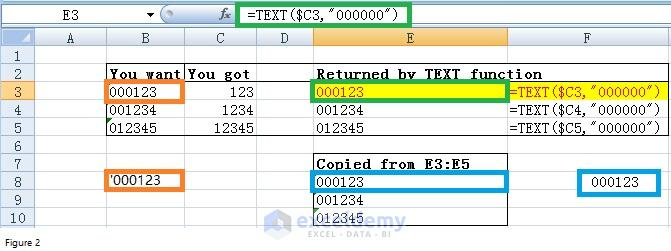Figure 2

## 3. Large number formatted as scientific notation

Any large number entered into Excel is stored as a number and abbreviated in scientific notation. Suppose that you put a long number like 12409003888123 in cell B2. Excel will apply a general number to it and the 12409003888123 will be presented in scientific notation as 1.2409E+13. Is it possible to prevent EXCEL from doing this?

The simplest way is to right click on cell B2. Then select Format Cells to open Format Cells dialog box. In the prompted Format Cells dialog box, select Number and set the number of decimal places as 0 (Figure 3). So far, the long number will be displayed as 12409003888123 instead of scientific notation.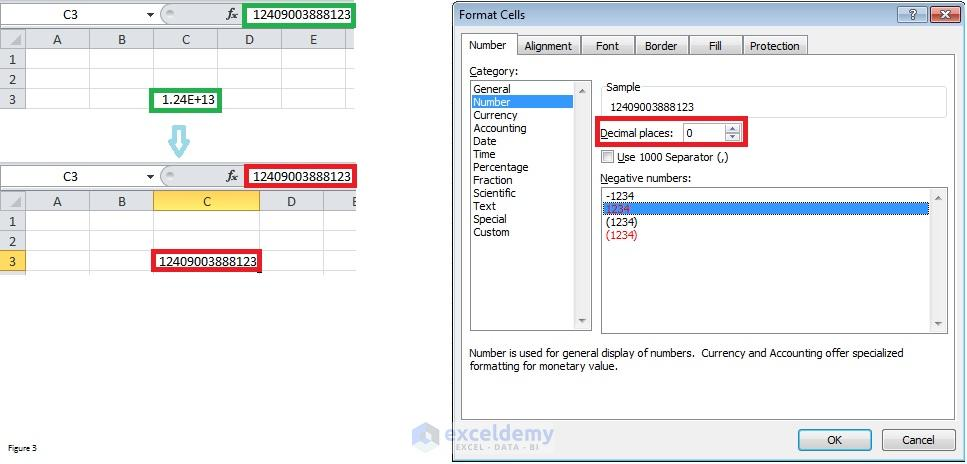Figure 3

## 4. VLOOKUP approximate match is “horrible”

Many people complain that you never be sure what VLOOKUP does with “approximate match”. Look at Figure 4.1, if your lookup value is “chun”, Excel will return \$93,500. When comparing against data table in range B2:E10, you will find that this value is not what we want. In our impression, the correct value should be \$151,200 as “chun” is similar to “Chung”.

Well, VLOOKUP approximate match does not work in the way that we think. It moves through the lookup table row by row and ultimately stops on the row in which the value is less than or equal to the lookup value when the value in the next row is greater than lookup value.

Let’s look at the above example again. “Bueller” is less than “chun” while “Chung” is greater than “chun” and therefore Excel stopped on 4th row and returned value \$93,500 in column C. Similarly, Excel stopped on 1st row after it reached value \$71,900 which is less than \$89,450 and the value in the next row is greater than \$89,450. The intersection of 1st row and column D gives you a commission rate of 6%.

Read More: How to Make an Excel Add-in [Narrated with an Example]

However, if you look at the data range closely, you will find that the commission rate for \$89,450 is %7. This is different from %6 that we just got from Figure 4.1. Is there anything wrong? Yes, we need to sort lookup table ascendingly first before using approximate match since VLOOKUP walks down the lookup table row by row.

Figure 4.2 shows another lookup table in range B12:E20. This table was sorted by Sales in ascending sequence. Now you will find that the commission rate for \$89,450 is correct now.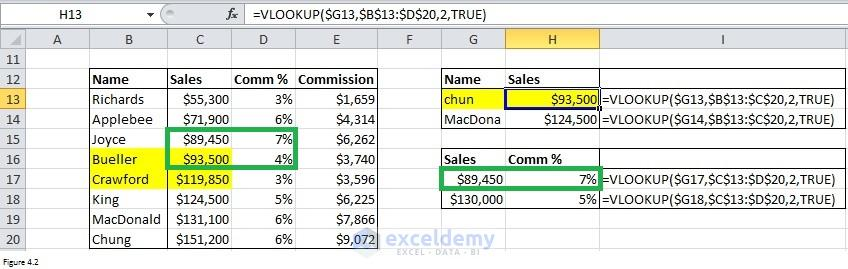Figure 4.2

Finally, I have to remind you again. Please sort the lookup range in ascending order before using VLOOKUP approximate match. Otherwise, you will get errors.

## 5. VLOOKUP is not case-sensitive

Many people are not aware that VLOOKUP is not case-sensitive and this may cause you to make mistake. Let’s look at Figure 5.1. “marie” will be matched when the VLOOKUP value is “Marie” and thus EXCEL returns 14 in cell F3. In fact, 23 in cell C4 is what we really want. How to prevent this kind of mistake from happening?

A combination of INDEX, MATCH, and EXACT functions can be used here to get correct number 23. RangeG8:G10 gives you formula returning numbers in range F8:F10. Formula “MATCH(TRUE,INDEX(EXACT(\$E8,\$B\$2:\$B\$10),0),0)” is the key to solving the problem. It gives you the row reference in which the exact match (Marie in our case) exists.  Have a try on your own. You will find that the row number is 3.

Another easier approach is to use the combination of OFFSET and MATCH function. For more details on OFFSET function, you can read one of my previous articles – Excel Offset Function with 3 Real Life Uses.

## 6. Cannot start from an arbitrary column or row with VLOOKUP

It is annoying that we can only perform a left-to-right lookup with VLOOKUP function.  But if you have read the article recommended in section 5, you will know that OFFSET/MATCH function can enable you starting the search from any column or row. In the following example, the value in cell C8 will change as you change the values in cell C7 and B8.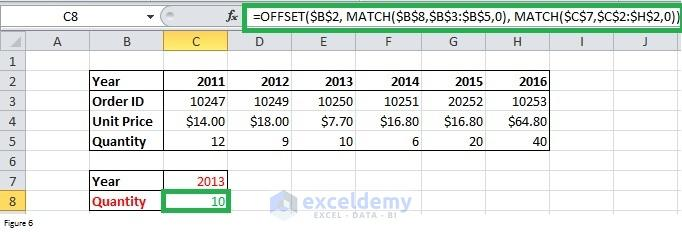Figure 6

## 7. VLOOKUP does not adjust column offset if you delete columns

Suppose that we have a lookup range starts from K3 through N10. And we do VLOOKUP approximate match in range P2:R4. Let ‘s take formula in cell Q3 as an example. The formula will be “=VLOOKUP(P3,K3:N10,3,TRUE)” if we want to retrieve approximate commission rate for “chun”.

After removing column L, you will find that the above formula will be replaced with “=VLOOKUP(O3,K3:M10,3,TRUE)”. The lookup table was changed automatically. Even the cell reference for lookup value was also changed from “P3” to “O3”. All of these are right. But the column offset is still 3. In fact, it should be 2 as commission rate is in the second column of the lookup table now.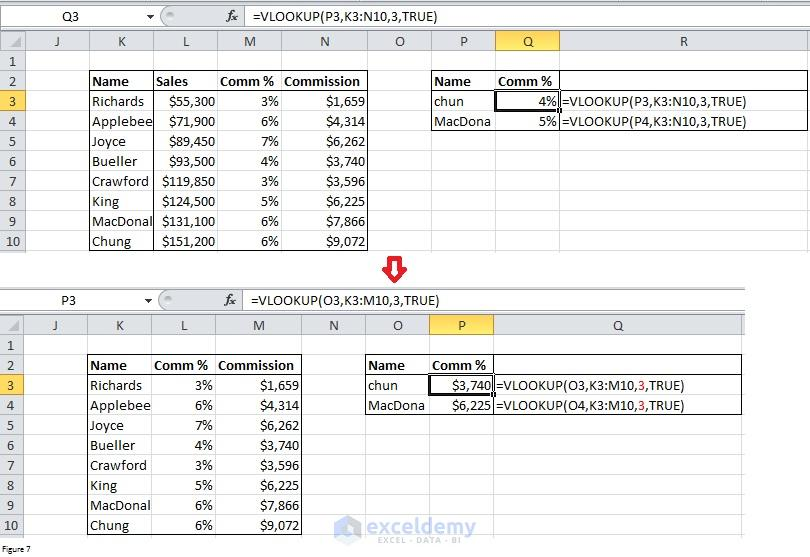Figure 7

## 8. Cell referencing changes when copying formulas

Look at Figure 8, you will see that the cell references will change after you copy formula “=COUNTIFS(\$C3:\$C10,”=”&\$F3)” from cell G3 to cell G4. The formula is “=COUNTIFS(\$C4:\$C11,”=”&\$F4)”  in cell G4 and this formula returns 4. But if you look at range B2:D10 closely, you will find that the number of male students should be 5 instead of 4. We got a mistake here. The reason is that you apply relative reference – \$C3:\$C10 and it can change after you copy it to another cell. The right way here is to apply absolute reference and replace “\$C3:\$C10” with “\$C4:\$C11”. Just like what we did in range F7:H9. It’s very easy to make errors when pasting formulas and you should be careful.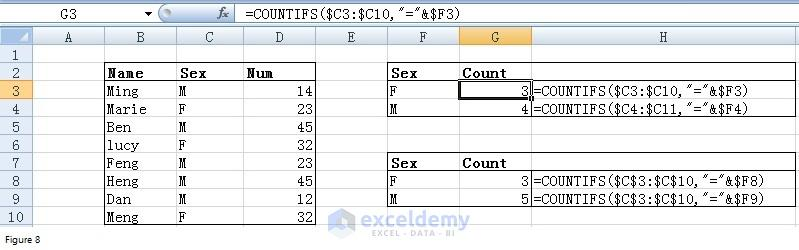Figure 8

## 9. Not easy to view whole content if the cell’s content is too long

In order to illustrate this issue, I just copied a paragraph from this article into cell B2. Figure 9.1 shows you that there is only one line in the worksheet and it is difficult to view the whole content.

After adding line break inside cell B2 by pressing ALT+ENTER, we can get something like that shown in Figure 9.2. There are 7 lines now.

## 10. Cannot work seamlessly with Microsoft Word

Excel splits one row containing a “carriage return” into multiple rows when copying a table from word into excel. Suppose that there is a doc file – Copy table from word to excel.docx – containing below table.

 No Details Date 1 Notification Date 22.07.2013 2 Last Date for Receiving offilled in Application Form 10.08.2013 3 Announcement of Eligible Candidates List for Entrance Test 13.08.2013

If we copy this table into a worksheet using CTRL+C and CTRL+V, we will get something like that shown in Figure 10.1. You can see that “Last Date for Receiving of filled in Application Form” is split into two rows. This is absolutely not what we want.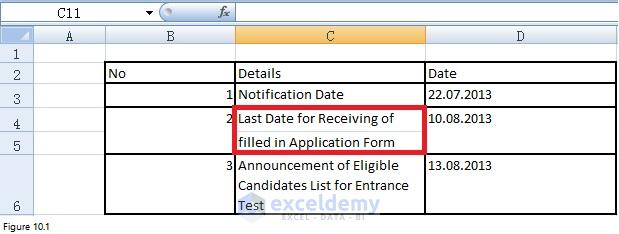Figure 10.1

To prevent this from happening, we can use VBA macro to extract tables from Word file into Excel file. The code in the following table can retrieve data from all the tables within a word document into Excel file.

 Source code```Sub Import_Table()

Dim wdDoc As Object

Dim wdFileName As Variant

Dim tableNo As Integer   'table number in Word

Dim iRow As Long    'row index in Excel

Dim iCol As Integer    'column index in Excel

Dim resultRow As Long

Dim tableStart As Integer

Dim tableTot As Integer

On Error Resume Next

ActiveSheet.Range("A:AZ").ClearContents

wdFileName = Application.GetOpenFilename("Word files (*.docx),*.docx", , _

"Browse for file containing table to be imported")

If wdFileName = False Then Exit Sub '(user canceled import file browser)

Set wdDoc = GetObject(wdFileName) 'open Word file

With wdDoc

tableNo = wdDoc.tables.Count

tableTot = wdDoc.tables.Count

If tableNo = 0 Then

MsgBox "This document contains no tables", _

vbExclamation, "Import Word Table"

ElseIf tableNo > 1 Then

tableNo = InputBox("This Word document contains " & tableNo & " tables." & vbCrLf & _

"Enter the table to start from", "Import Word Table", "1")

End If

resultRow = 1

For tableStart = 1 To tableTot

With .tables(tableStart)

'copy cell contents from Word table cells to Excel cells

For iRow = 1 To .Rows.Count

For iCol = 1 To .Columns.Count

ThisWorkbook.Worksheets("Import_Table").Cells(resultRow, iCol) = WorksheetFunction.Clean(.cell(iRow, iCol).Range.Text)

Next iCol

resultRow = resultRow + 1

Next iRow

End With

resultRow = resultRow + 1

Next tableStart

End With

End Sub```

After clicking on the Import table button, we will get a table similar to that in Figure 10.2. I changed the column width here manually to demonstrate that “Last Date for Receiving of filled in Application Form” was not split into two rows.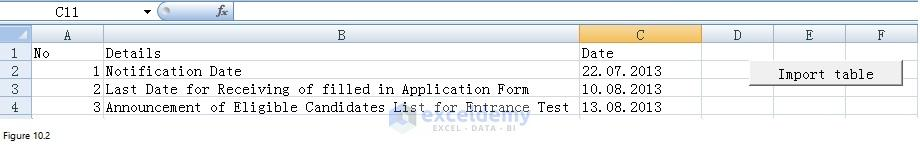Figure 10.2

## 11. Recalculation of formulas takes a long time sometimes

Excel recalculates your workbook’s formulas when you open the workbook or when you make significant changes. This feature is good but these recalculations can take a long time. It is not so good from this point of view.  Below are two approaches for you to control calculation and save your time.

### Turn off automatic recalculation

Click on the File tab -> Options and then click the Formulas category.  You will get Excel Options dialog box as shown in Figure 11.1. “Automatic” under Workbook Calculation in the Calculation options section means that Excel will recalculate all dependent formulas every time you make a change to a value, formula or a name. “Automatic except for data tables” means that Excel will recalculate all dependent formulas except for data tables. The last choice “Manual” can enable you to turn off automatic recalculation.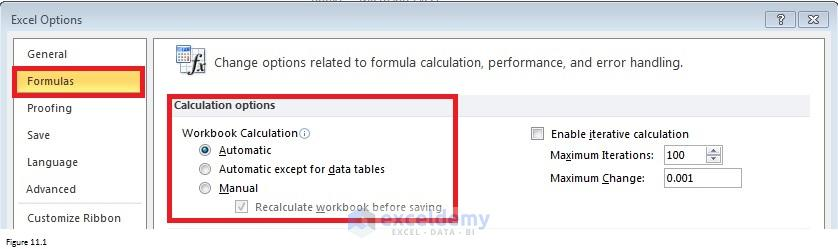Figure 11.1

Figure 11.2 presents you another method to turn off automatic recalculation. There are also three options for you to choose: Automatic, Automatic Except for Data Tables and Manual.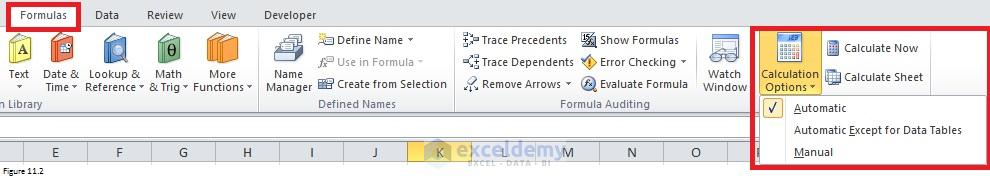Figure 11.2

After you turn off automatic recalculation, you can click the Calculate Now button in the Calculation group on the Formulas tab (Figure 11.2). This method can enable Excel to recalculate all open worksheets. Another option – Calculate Sheet button (also shown in Figure 11.2) – can make Excel recalculate active worksheet and any charts/chart sheets linked to this worksheet.

### Change the number of times Excel iterates a formula

Please make sure Enable iterative calculation check box was selected before you set maximum iterations and maximum change.  The higher the number of iterations, the more time Excel will need to recalculate a worksheet. The smaller the maximum amount of change, the more accurate the result and the more time Excel needs to recalculate a worksheet.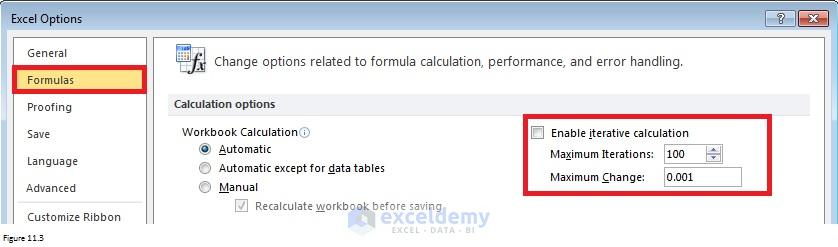Figure 11.3

## 12. No easy way to rename multiple worksheets

Sometimes, we need to insert multiple worksheets. What will you do to rename all of those worksheets?  Use Rename command to rename them one by one? What if you have to rename 100 worksheets? It will take you a lot of time to finish the task. Luckily, we can use VBA code for renaming multiple worksheets.

Here is the code for inserting and rename multiple worksheets.

 Source code```Sub rename_tab()

'Define variable

Dim wbk As Workbook

Dim ws As Worksheet

'Open workbookFile

Set fnm = ThisWorkbook.Sheets(1).Cells(1, 2)

Set wbk = Workbooks.Open(fnm)

'Set workbook as active workbook

wbk.Activate

For i = 2 To ThisWorkbook.Worksheets(2).UsedRange.Rows.Count

'Return number of all worksheet in the active workbook

n = Worksheets.Count

'Add worksheet after the last worksheet

'Give the newly added worksheet a name

ws.Name = ThisWorkbook.Worksheets(2).Cells(i, 2)

Next i

'Close and save active workbook

wbk.Close Savechanges:=True

End Sub```

Figure 12.1 shows you how to design and use this macro. Suppose that the macro was saved in Insert and Rename Multiple Tabs.xlsm files. There are two tabs in this xlsm file. The first one (left panel of Figure 12.1) is to include file in which you want to insert tabs. You need to enter the file pathname into cell B1. The macro will open the file listed in cell B1 (WS.xlsx in our case). The second worksheet (right panel of Figure 12.1) provides tab names which will be used by VBA macro. You can add as many as tab names into the second worksheet.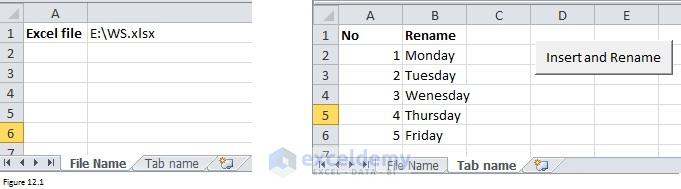Figure 12.1

Figure 12.2 presents you the screenshots for WS.xlsx before and after running above VBA code.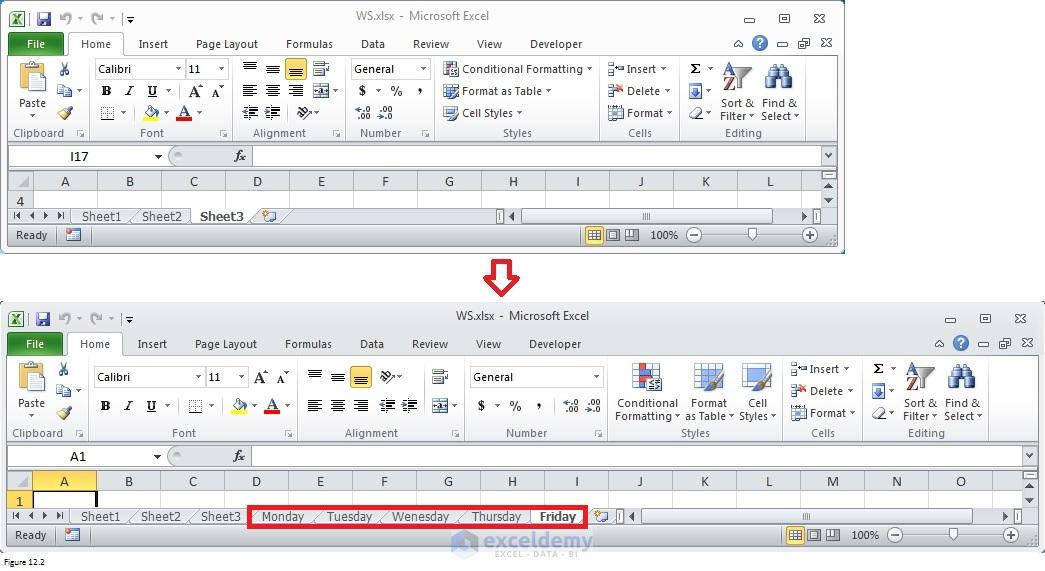Figure 12.2

## 13. Pivot tables do not have median

A pivot table is one of the most powerful features. It allows you to summarize, analyze, explore and present your data. It helps you extract the significant insigt froinsightrge and detailed data set.

The left panel in Figure 13.1 contains data to be analyzed in our case. You can see that we have height and weight for different students as well as their sex and age. Click any cell in the range B2:F21. On the Insert tab, click PivotTable. Choose the default location in the prompted Create PivotTable dialog box. Range H2:L21 in the same worksheet was chosen in this case. You can see that this range is selected in the middle panel of Figure 13.1. In the appeared PivotTable field list dialog box (right panel of Figure 13.1), drag SEX and AGE to the Row Labels area. Then drag WEIGHT to the Values area. Finally, a pivot table including cells from H2 through I16 was created.

Per the pivot table, the sum of weight for male students who are 11 is 57.5. And the sum of weight for female students who are 13 is 121.8. Plus the sum statistics, we can also count the number of students who are 13 or compute the average weight. Right-click on any cell in the pivot table (range H2:I16) and then choose Value Field Settings. In the Value Field Settings dialog box (right panel of Figure 13.2), you can select the type of calculation in which you are interested.

The type of calculation includes Count, Average, Max, Min, etc.  If you read through all types of calculation, you will notice that EXCEL does not provide with the Median statistics in the pivot table. Then what should we do if we really want to calculate median statistics? The MEDIAN function is a good option.

Before using MEDIAN function, it is better to sort data by SEX and AGE. The left panel in Figure 13.3 shows data copied from range B2:F21. And right panel in Figure 13.3 shows how the range looks after sorting.

Now let’s use MEDIAN function to calculate Median for each subgroup. Formula “=MEDIAN(E27:E35)” in cell K3 returns 62.5. It tells that the median weight for female students whose age is between 11 and 15 is 62.5 kg.

## 14. Pivot tables cannot count unique values

Sometimes, we many want to find out how many unique values exist in a range that contains duplicate values. But Pivot tables do not count unique values. Figure 14.1 illustrates on this.  You can find that there are 2 students who are 11 years old and 5 students who are 12 years old. But if you want to know the range of age distribution, you have to count one by one manually.

Fortunately, we have ROWS function which can return the number of rows in the reference range. By entering formula “=ROWS(H27:H23)” into cell H35, we can get 6. It tells that there are 6 age groups, covering from 11 to 16.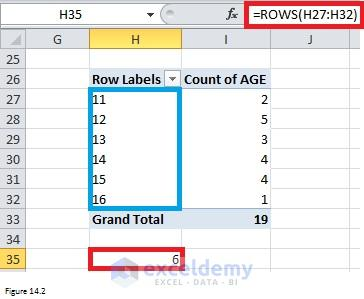Figure 14.2

And here Figure 14.3 shows you how to count unique values for different sex groups.  What we did here is to apply ROWS function to each subgroup. For the female group, enter formula “=ROWS(H4:H8)” into cell K3. And for the male group, enter formula “=ROWS(H10:H15)” into cell K9 and it will return 6.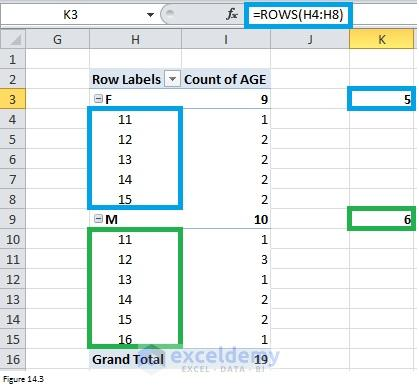Figure 14.3

## 15. No sumifs/countifs/averageifs equivalents for functions such as max or median

The SUMIFS function can sum cells meeting multiple criteria. SUMIF function can only apply one criterion while SUMIFS function can be applied on more than one set of criteria with more than one range. The syntax is SUMIFs(sum_range, criteria_range1, criteria1, [criteria_range2,criteria2,]….). Sum_range means the range to be summed. Criteria_range and criteria are provided in pairs. Let’s look at Figure 15.1. It has the same sample data as that in Section 13 (pivot table). You can compare this figure against Figure 13.1 to have a deep understanding. The sum range for cell I4 is E3:E21. The first criterion is “C3:C21 = F” while the second criterion is “D3:D21 = 11”. The sum of weights for female students who are 11 years old is 51.3 kg. This is the same as that calculated by Pivot table.

AVERAGEIFS function has similar syntax with SUMIFS. Figure 15.2 shows you how to use AVERAGEIFS function.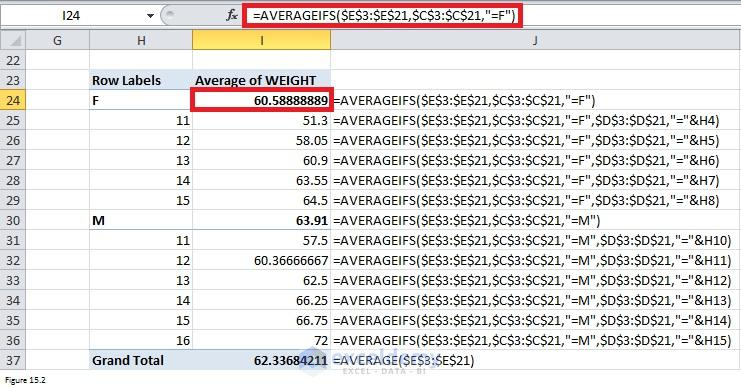Figure 15.2

COUNTIFS function is slightly different from SUMIFS function and AVERAGEIFs function.  The syntax only consists of different pairs of criteria_range and criteria. The range like sum_range or average_range should be removed. Figure 15.3 shows you how to apply COUNTIFs function.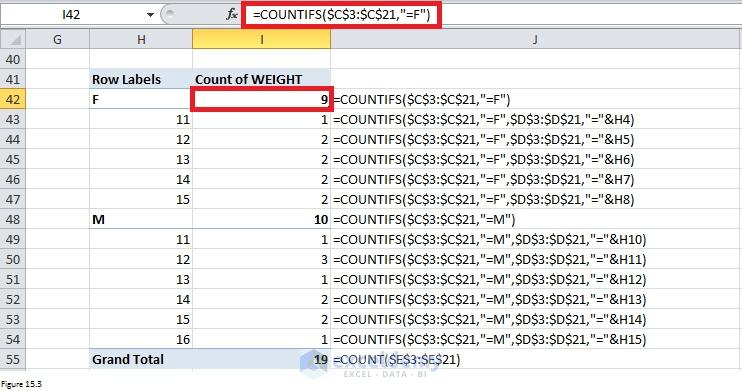Figure 15.3

These functions are useful, right? However, there is no equivalent function like MEDIANIFs function. To calculate Median for a subgroup, we can use the same approach in section 13 by sorting sample and then apply MEDIAN function. You can refer to section 13 for details.

## 16. Features available in Excel for Windows that are not available in Excel for Mac

Some useful reporting features do not work in Excel for Mac and thus you cannot use them when generating reports for clients who use Mac. Besides these reporting features, there are also other features that are incorporated into Windows version of Excel but are not included in Mac version of Excel.

For example, Excel for Windows allows you to set a default location for saving files. It can automatically save “draft” copies of your workbook as you work to minimize your loss if Excel crashes suddenly. Windows version of Excel can also allow you to customize Quick Access Toolbar. All these three features are not supported by Excel for Mac.

With Excel for Windows, you can preview workbook in three modes: Normal, Page Layout, and Page Break. But with Excel for Mac, you can only use Normal and Page Layout preview mode. As for the print preview, you can see a large print preview of workbook and zoom in or out in windows version of Excel. But for Excel in Mac, you can only see a small print preview which cannot be zoomed. The View Side by Side feature which allows you to compare two workbooks easily is only available in Windows version of Excel. And the Synchronous Scrolling that allows you to scroll through two workbooks at the same time is also missing in Excel for Mac.

There are so many features that I cannot include them all. For more details, you can read this article.

## 17. Cannot quick access to tables in Excel Options dialog box

If you open Excel Options dialog box, you will find that there are 10 tabs and each of them is filled with dozens of settings. Locating a specific can waste your considerable time. Figure 11.1 and Figure 11.2 are good illustrations for this issue.

## 18. Limits of Excel

It is well known that Excel has limits. For example, whether the workbook can be opened will be limited by available memory and system resources. The maximum number of rows is 1,048,576 and the maximum number of columns is 16, 384. Here is a summary of all Excel limits.

But some limitations are annoying. For instance, the maximum number precision is 15. It means that the number of digits a cell can contain is only 15. Excel will replace digits (after the 15th digit) with zeros. If you enter “12345678901234567890” into a cell, you will get “12345678901234500000” instead.

## 19. No Automatic spell checking in Excel

Microsoft Word offers automatic spell checking which can check the spelling and grammar of your files. It can highlight the mistakes. However, Excel does not automatically highlight the mistake. But using the Reviews -> Proofing -> Spelling command, you can check out the errors with your Excel files.

## 20. Excel Crashes easily when running macros

Perhaps you have the same experience that EXCEL just crashes when you are running the VBA macro, especially when you use DO WHILE loop or FOR NEXT loop which has to loop for a lot of times (like 1000 or 10,000 times).  I used to scrape data from the internet to extract name and contact information of more than 10,000 companies. It took me almost 24 hours in total to finish the work. Initially, it crashed after running the macro for 1 or 2 hours due to bad internet condition. Suddenly, all the work disappeared. I was so frustrated after it crashed the second time. It suddenly occurred to me that I can save the workbook once one loop is finished.  After adding the one statement “ThisWorkbook.Save” at the end of each loop, I have never lost any work due to excel crashing at the wrong time.

Use of Offset Function in Excel [Offset – Match Combo, Dynamic Range]

An Excel VBA function with one argument

VLOOKUP Function in Excel: Learn with Examples

How to Split Cells in Excel (The Ultimate Guide)

#### Zhiping Yan

I am from China and this photo was taken in a classical garden. There are many similar gardens in China, attracting a lot of visitors every year, especially in spring and summer. I was major in Biotechnology. But I took a job as an SAS programmer because I prefer programming. Besides SAS, I also learned Excel VBA in my spare time. It is fantastic to be able to manipulate data, files and even to interact with the internet via programming. This will save me a lot of time. I am keen to learn new things.